Publicité

# Vlsi gate level design

Postgraduate Student à GITAM DEEMED University
30 Mar 2020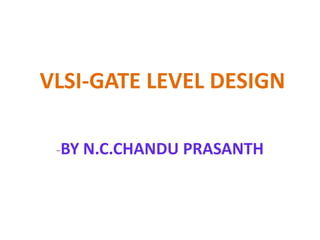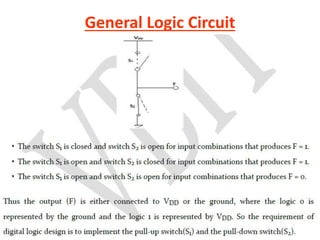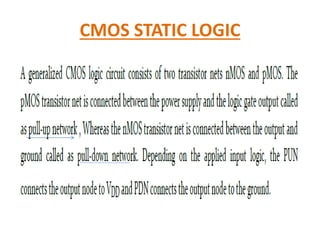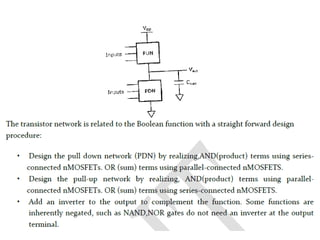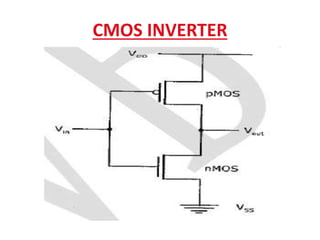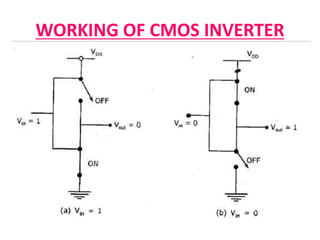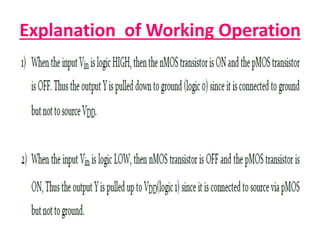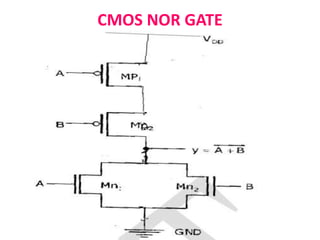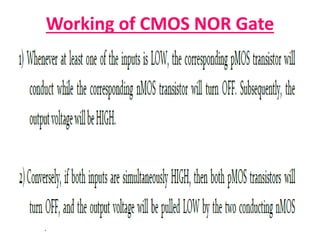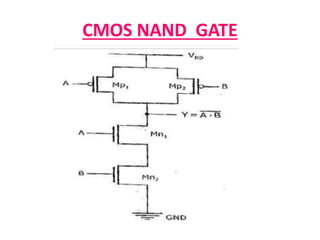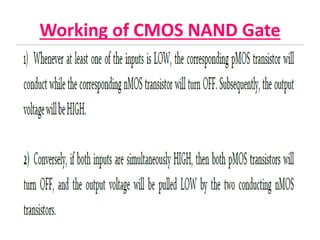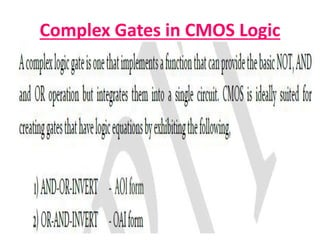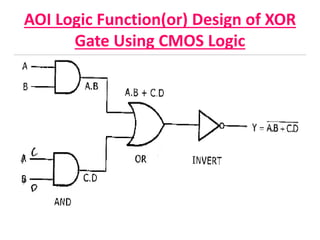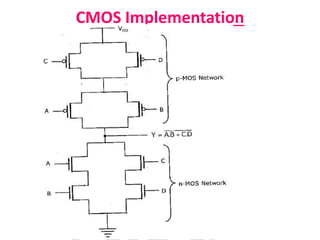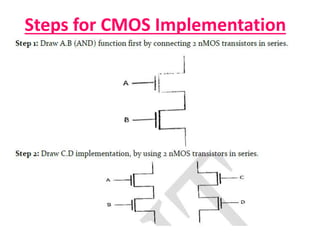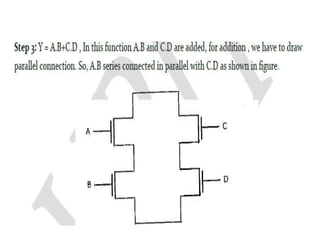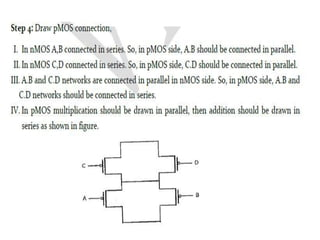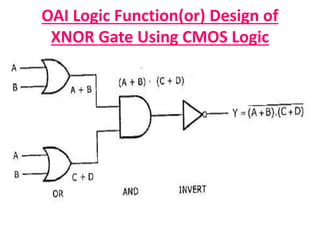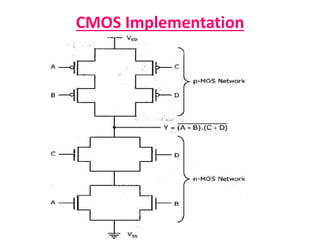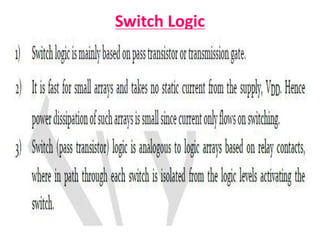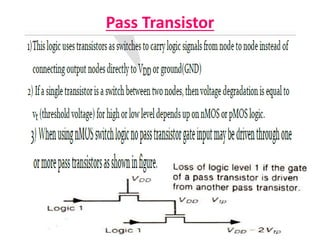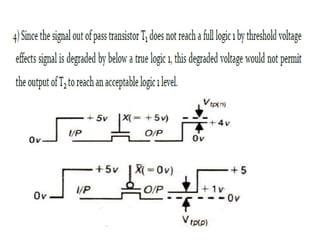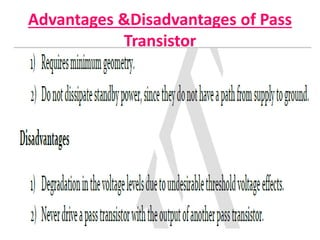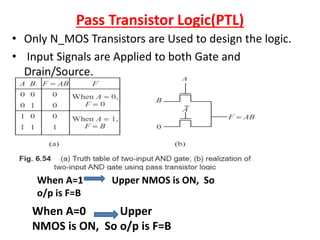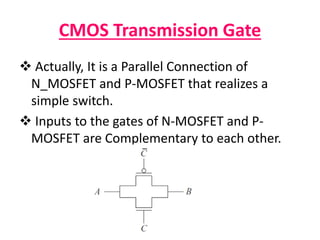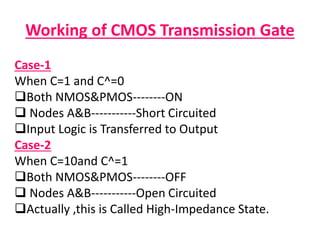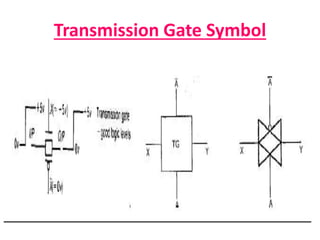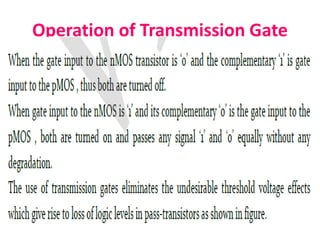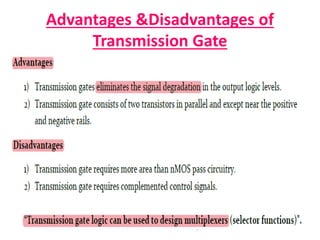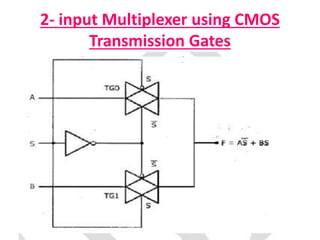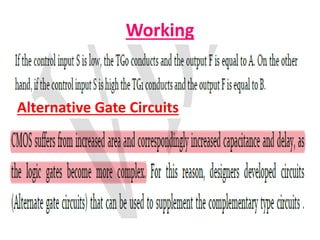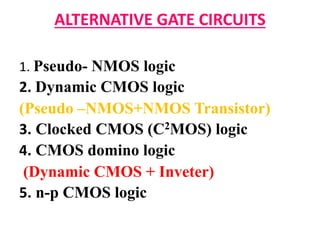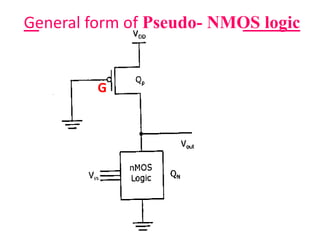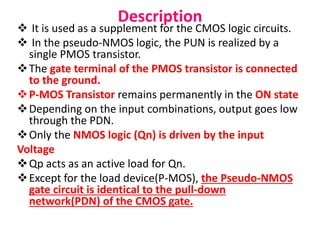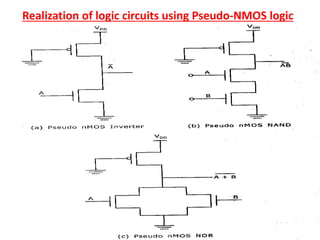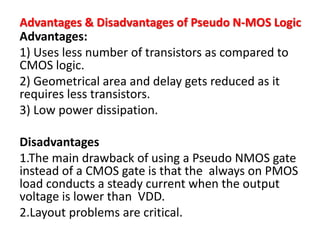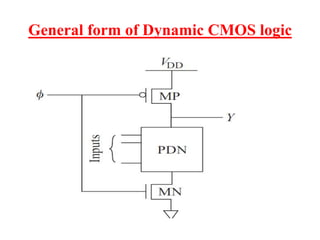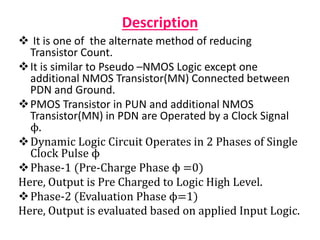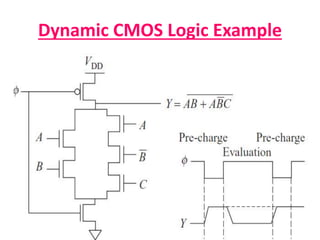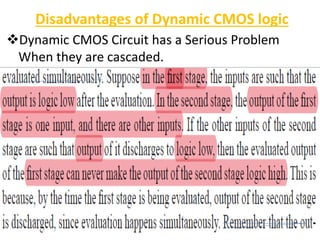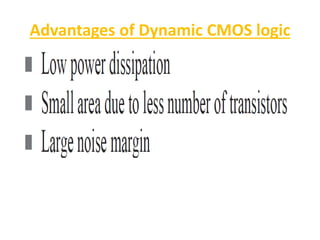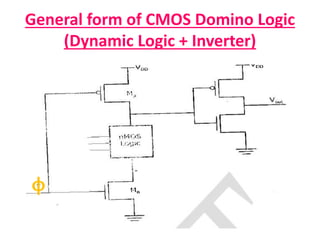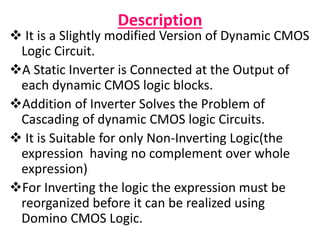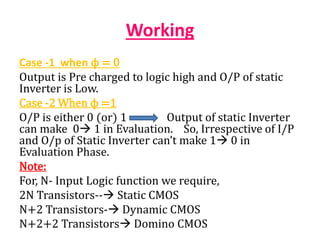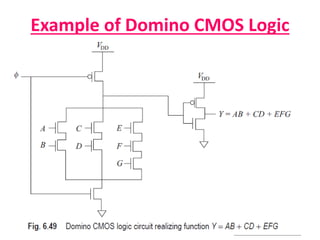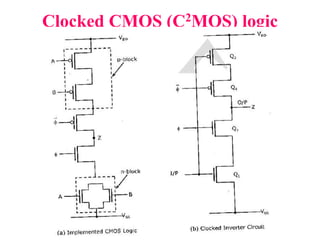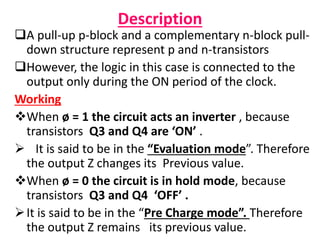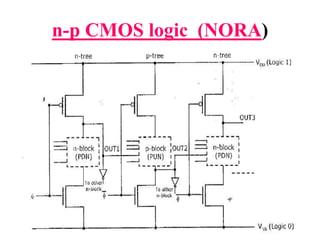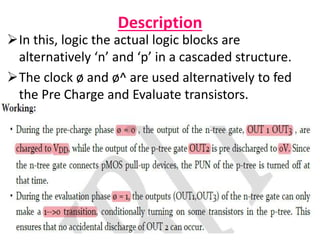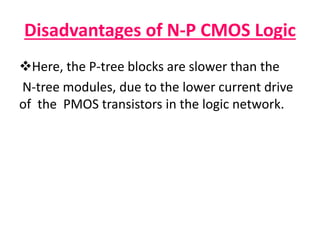1 sur 50
Publicité

### Vlsi gate level design

1. VLSI-GATE LEVEL DESIGN -BY N.C.CHANDU PRASANTH
2. General Logic Circuit General Logic Circuit:
3. CMOS STATIC LOGIC
4. CMOS INVERTER
5. WORKING OF CMOS INVERTER
6. Explanation of Working Operation
7. CMOS NOR GATE
8. Working of CMOS NOR Gate
9. CMOS NAND GATE
10. Working of CMOS NAND Gate
11. Complex Gates in CMOS Logic
12. AOI Logic Function(or) Design of XOR Gate Using CMOS Logic
13. CMOS Implementation
14. Steps for CMOS Implementation
15. OAI Logic Function(or) Design of XNOR Gate Using CMOS Logic
16. CMOS Implementation
17. Switch Logic
18. Pass Transistor
20. Pass Transistor Logic(PTL) • Only N_MOS Transistors are Used to design the logic. • Input Signals are Applied to both Gate and Drain/Source. When A=1 Upper NMOS is ON, So o/p is F=B When A=0 Upper NMOS is ON, So o/p is F=B
21. CMOS Transmission Gate  Actually, It is a Parallel Connection of N_MOSFET and P-MOSFET that realizes a simple switch.  Inputs to the gates of N-MOSFET and P- MOSFET are Complementary to each other.
22. Working of CMOS Transmission Gate Case-1 When C=1 and C^=0 Both NMOS&PMOS--------ON  Nodes A&B-----------Short Circuited Input Logic is Transferred to Output Case-2 When C=10and C^=1 Both NMOS&PMOS--------OFF  Nodes A&B-----------Open Circuited Actually ,this is Called High-Impedance State.
23. Transmission Gate Symbol
24. Operation of Transmission Gate
26. 2- input Multiplexer using CMOS Transmission Gates
27. Working Alternative Gate Circuits
28. ALTERNATIVE GATE CIRCUITS 1. Pseudo- NMOS logic 2. Dynamic CMOS logic (Pseudo –NMOS+NMOS Transistor) 3. Clocked CMOS (C2MOS) logic 4. CMOS domino logic (Dynamic CMOS + Inveter) 5. n-p CMOS logic
29. General form of Pseudo- NMOS logic G
30. Description  It is used as a supplement for the CMOS logic circuits.  In the pseudo-NMOS logic, the PUN is realized by a single PMOS transistor. The gate terminal of the PMOS transistor is connected to the ground. P-MOS Transistor remains permanently in the ON state Depending on the input combinations, output goes low through the PDN. Only the NMOS logic (Qn) is driven by the input Voltage Qp acts as an active load for Qn. Except for the load device(P-MOS), the Pseudo-NMOS gate circuit is identical to the pull-down network(PDN) of the CMOS gate.
31. Realization of logic circuits using Pseudo-NMOS logic
32. Advantages & Disadvantages of Pseudo N-MOS Logic Advantages: 1) Uses less number of transistors as compared to CMOS logic. 2) Geometrical area and delay gets reduced as it requires less transistors. 3) Low power dissipation. Disadvantages 1.The main drawback of using a Pseudo NMOS gate instead of a CMOS gate is that the always on PMOS load conducts a steady current when the output voltage is lower than VDD. 2.Layout problems are critical.
33. General form of Dynamic CMOS logic
34. Description  It is one of the alternate method of reducing Transistor Count. It is similar to Pseudo –NMOS Logic except one additional NMOS Transistor(MN) Connected between PDN and Ground. PMOS Transistor in PUN and additional NMOS Transistor(MN) in PDN are Operated by a Clock Signal ϕ. Dynamic Logic Circuit Operates in 2 Phases of Single Clock Pulse ϕ Phase-1 (Pre-Charge Phase ϕ =0) Here, Output is Pre Charged to Logic High Level. Phase-2 (Evaluation Phase ϕ=1) Here, Output is evaluated based on applied Input Logic.
35. Dynamic CMOS Logic Example
36. Disadvantages of Dynamic CMOS logic Dynamic CMOS Circuit has a Serious Problem When they are cascaded.
37. Advantages of Dynamic CMOS logic
38. General form of CMOS Domino Logic (Dynamic Logic + Inverter) ϕ
39. Description  It is a Slightly modified Version of Dynamic CMOS Logic Circuit. A Static Inverter is Connected at the Output of each dynamic CMOS logic blocks. Addition of Inverter Solves the Problem of Cascading of dynamic CMOS logic Circuits.  It is Suitable for only Non-Inverting Logic(the expression having no complement over whole expression) For Inverting the logic the expression must be reorganized before it can be realized using Domino CMOS Logic.
40. Working Case -1 when ϕ = 0 Output is Pre charged to logic high and O/P of static Inverter is Low. Case -2 When ϕ =1 O/P is either 0 (or) 1 Output of static Inverter can make 0 1 in Evaluation. So, Irrespective of I/P and O/p of Static Inverter can’t make 1 0 in Evaluation Phase. Note: For, N- Input Logic function we require, 2N Transistors-- Static CMOS N+2 Transistors- Dynamic CMOS N+2+2 Transistors Domino CMOS
41. Example of Domino CMOS Logic
42. Clocked CMOS (C2MOS) logic
43. Description A pull-up p-block and a complementary n-block pull- down structure represent p and n-transistors However, the logic in this case is connected to the output only during the ON period of the clock. Working When ø = 1 the circuit acts an inverter , because transistors Q3 and Q4 are ‘ON’ .  It is said to be in the “Evaluation mode”. Therefore the output Z changes its Previous value. When ø = 0 the circuit is in hold mode, because transistors Q3 and Q4 ‘OFF’ . It is said to be in the “Pre Charge mode”. Therefore the output Z remains its previous value.
44. n-p CMOS logic (NORA)
45. Description In this, logic the actual logic blocks are alternatively ‘n’ and ‘p’ in a cascaded structure. The clock ø and ø^ are used alternatively to fed the Pre Charge and Evaluate transistors.
46. Disadvantages of N-P CMOS Logic Here, the P-tree blocks are slower than the N-tree modules, due to the lower current drive of the PMOS transistors in the logic network.
Publicité